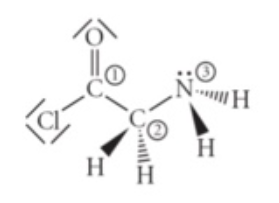# Problem: Consider the molecule below. Determine the molecular geometry at each of the 3 labeled atoms. A) 1 = trigonal planar, 2 = tetrahedral, 3=tetrahedral B) 1 = trigonal planar, 2 = trigonal pyramidal, 3 = trigonal pyramidal C) 1 = tetrahedral, 2 = tetrahedral, 3 = tetrahedral D) 1 = tetrahedral, 2 = tetrahedral, 3 = trigonal planar E) 1 = trigonal planar, 2 = tetrahedral, 3 = trigonal pyramidal

###### FREE Expert Solution100% (315 ratings)###### Problem Details

Consider the molecule below. Determine the molecular geometry at each of the 3 labeled atoms.A) 1 = trigonal planar, 2 = tetrahedral, 3=tetrahedral

B) 1 = trigonal planar, 2 = trigonal pyramidal, 3 = trigonal pyramidal

C) 1 = tetrahedral, 2 = tetrahedral, 3 = tetrahedral

D) 1 = tetrahedral, 2 = tetrahedral, 3 = trigonal planar

E) 1 = trigonal planar, 2 = tetrahedral, 3 = trigonal pyramidal